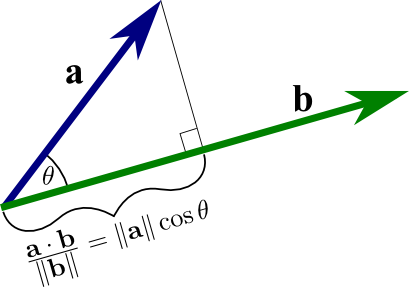# Math Insight

### Image: Dot product as projection of vectorsThe dot product of vectors $\vc{a}$ and $\vc{b}$, when divided by the magnitude of $\vc{b}$, is the projection of $\vc{a}$ onto $\vc{b}$, i.e., $$\frac{\vc{a}\cdot \vc{b}}{\|\vc{b}\|} = \|\vc{a}\| \cos\theta,$$ where $\theta$ is the angle between $\vc{a}$ and $\vc{b}$.

Image file: dot_product_projection.png

Source image file: dot_product_projection.svg
Source image type: Inkscape SVG